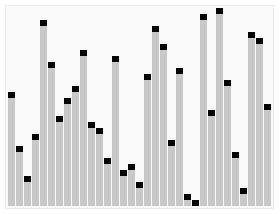# Algorithm - Complexity (Big O)

Complexity is based off of how the algorithm scales for very large n.

Constants and lower-order terms are dropped.

For example:

• $O(1)$ will execute in constant time
• a model that takes $100*n^2$ time has $O(n^2)$ complexity,
• a model that takes $4n + n^2$ time has also $O(n^2)$ complexity.

A counterexample for this question is:

• Algorithm A has running time $n^3$ and algorithm B has running time $10n^2$ . Until $n >= 10$ , A is faster than B.

## order N

• 40 records, 40 comparisons
• N records, N Comparison
• The algorithmic complexity is order N: O(N)

Linear time Algorithm

• 40 records, 4 comparisons
• N records, log(N) Comparisons

The algorithmic complexity is order: O(log(N)). Far better scalable than the previous.

In a relational database, you get this logarithm access time pattern through the access of a sequence.

## Parallel Workers

In a read Trimming (trim function), we can't use an index. We have to touch every record no matter what.

We can do better with parallel tasks (of workers)

• For 40 records
• with 7 workers
• we need 7 (Cycles|Time) to trim all records

The algorithmic complexity is order: O(N/k)

N is the number of items to operate on and k is the number of workers. Because the execution is parallel, the amount of time it takes to complete the task (time complexity) is number of items to operate on divided by the number of workers.

Discover MoreAlgorithm - Big O Notation

The big-Oh notation is a vocabulary for the design and analysis of algorithms. See: Asymptotic analysis Notation is just . Terminology: running time is O(n...Ordinal Data - Sorting (Problem / Algorithm)

Input: array of n numbers, unsorted. Output: array of n numbers, sorted (from smallest to largest) Possible Assumption: numbers are distinct with duplicates, the problem can even be easier Sorting...